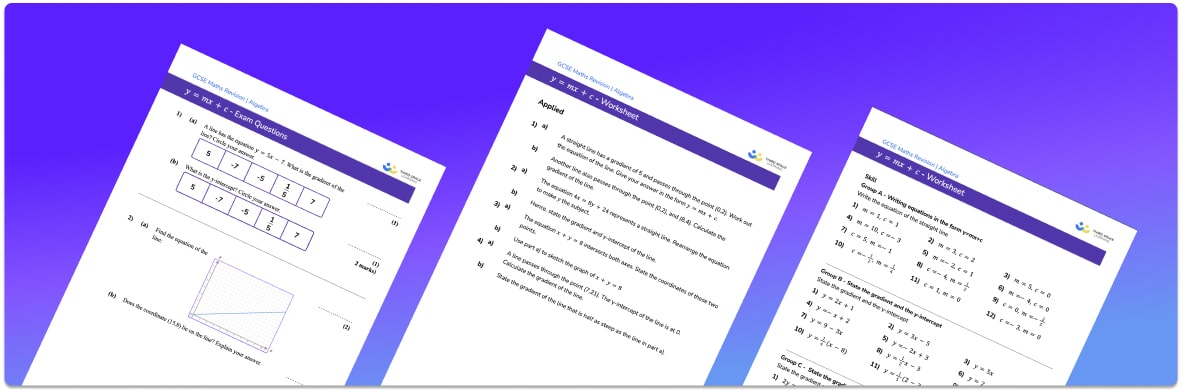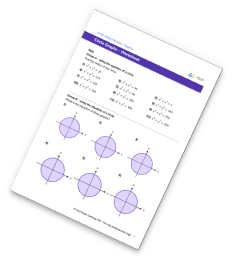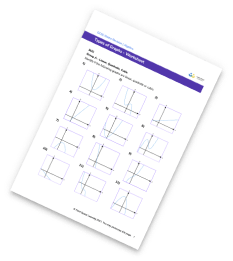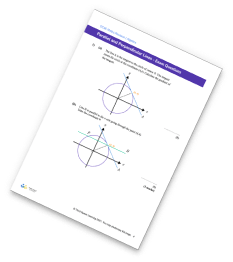# Y=Mx+C WorksheetHelp your students prepare for their Maths GCSE with this free y=mx+c worksheet of 30+ questions and answers

• Section 1 of the y=mx+c worksheet contains 20+ skills-based y=mx+c questions, in 3 groups to support differentiation
• Section 2 contains 3 applied y=mx+c questions with a mix of worded problems and deeper problem solving questions
• Section 3 contains 3 foundation and higher level GCSE y=mx+c  exam questions
• Answers and a mark scheme for all y=mx+c questions are provided
• Questions follow variation theory with plenty of opportunities for students to work independently at their own level
• All questions created by fully qualified expert secondary maths teachers
• Suitable for GCSE maths revision for AQA, OCR and Edexcel exam boards
• To receive this resource and regular emails with more free resources, blog posts and other Third Space updates, enter your email address and click below.

• This field is for validation purposes and should be left unchanged.

You can unsubscribe at any time (each email we send will contain an easy way to unsubscribe). To find out more about how we use your data, see our privacy policy.

### Y=Mx+C at a glance

y=mx+c is the general equation of a straight line. When written in the form y=mx+c, m is the gradient of the line and c is the y intercept. The gradient of a straight line tells us how steep the line is and the bigger the gradient, the steeper the line. A line with a negative gradient slopes downwards. The y intercept of the line tells us the y coordinate of the point where the line passes through the y axis.

Sometimes the equations of linear graphs may be written in a form other than y=mx+c. If this is the case, we can rearrange the equation into the form y=mx+c, i.e. make y the subject, to give us the gradient and y intercept of the line.

If we have a straight line graph, we can find the equation of the straight line by finding the gradient and the y intercept of the line and substituting these values into the equation y=mx+c. To find the gradient, we need to identify the x coordinates and y coordinates of two points, subtract the y values, subtract x values and then divide them.

Looking forward, students can then progress to additional algebra worksheets, for example a simplifying expressions worksheet or simultaneous equations worksheet.For more teaching and learning support on Algebra our GCSE maths lessons provide step by step support for all GCSE maths concepts.

## Related worksheets

Circle Graph WorksheetParallel And Perpendicular Lines WorksheetGraph Worksheet## Do you have KS4 students who need more focused attention to succeed at GCSE?There will be students in your class who require individual attention to help them succeed in their maths GCSEs. In a class of 30, it’s not always easy to provide.

Help your students feel confident with exam-style questions and the strategies they’ll need to answer them correctly with our dedicated GCSE maths revision programme.

Lessons are selected to provide support where each student needs it most, and specially-trained GCSE maths tutors adapt the pitch and pace of each lesson. This ensures a personalised revision programme that raises grades and boosts confidence.

Find out more